# 第五章 Q-learning 和 贝尔曼方程

$V(s)=\mathbb{E}\left[\sum_{t=0}^{\infty} r_{t} \gamma^{t}\right]$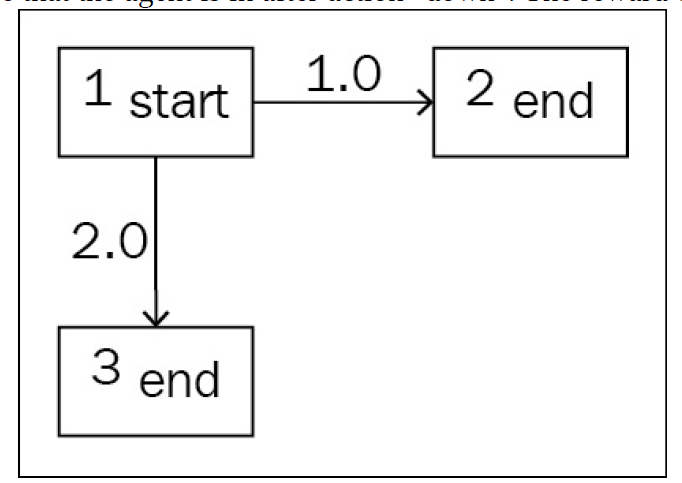• 我们的初始状态是 1：start。
• 往右走到达终点状态2，并获得reward 1。
• 往下走到达终点状态3； 并获得reward 2。

• Agent无脑往右， 到达状态2。 那么Value 就是 1.
• Agent无脑往下， 到达状态3。 那么Value 就是 2.
• Agent 有 50%的概率往右，50%概率往下。 根据Value的计算公式， 此时应该求取期望， 即$0.5\times 1 + 0.5 \times 2 = 1.5$
• Agent 有 10%的概率往右，90%概率往下。 根据Value的计算公式， 此时应该求取期望， 即$1.5\times 1 + 0.9 \times 2 = 1.9$

## 贝尔曼方程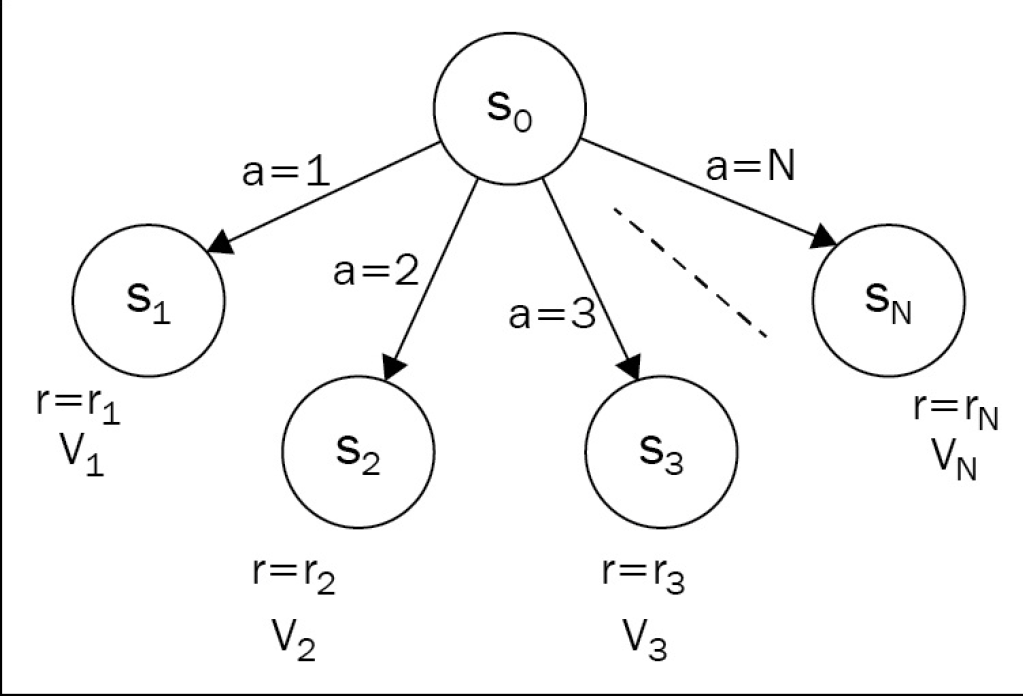$V_1, V_2, ...$就是上一节讨论的 $s_1, s_2$状态所对应的 Value。
$V_0$代表我们想要探究的当前状态$s_0$的Value：

$V_{0}=\max _{a \in 1 \ldots N}\left(r_{a}+\gamma V_{a}\right)$

$S$代表$a$动作可能得到的状态集合。 $p_{a, 0\rightarrow s}$代表转移概率。

$V_{0}=\max _{a \in A} \mathbb{E}_{s \sim S}\left[r_{s, a}+\gamma V_{s}\right]=\max _{a \in A} \sum_{s \in S} p_{a, 0 \rightarrow s}\left(r_{s, a}+\gamma V_{s}\right)$

## Value of Action 动作的价值

$Q_{s,a}$的定义基于我们刚刚对State Value的定义：
$Q_{s, a}=\mathbb{E}_{s^{\prime} \sim S}\left[r_{s, a}+\gamma V_{s^{\prime}}\right]=\sum_{s^{\prime} \in S} p_{a, s \rightarrow s^{\prime}}\left(r_{s, a}+\gamma V_{s^{\prime}}\right)$

$V_{s}=\max _{a \in A} Q_{s, a}$

$Q(s, a)=r_{s, a}+\gamma \max _{a^{\prime} \in A} Q\left(s^{\prime}, a^{\prime}\right)$

### 一个简单的例子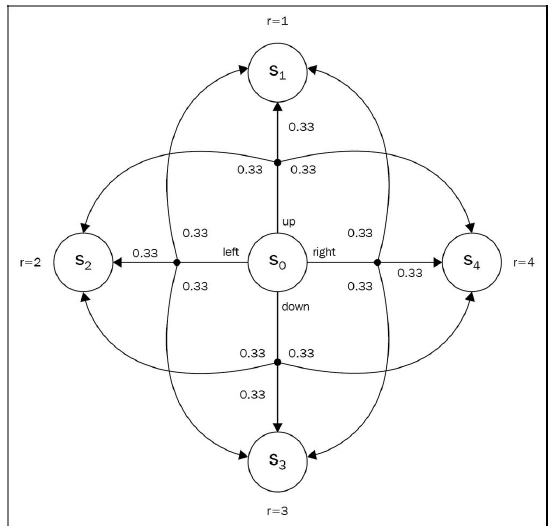$Q_{s, a}=\mathbb{E}_{s^{\prime} \sim S}\left[r_{s, a}+\gamma V_{s^{\prime}}\right]=\sum_{s^{\prime} \in S} p_{a, s \rightarrow s^{\prime}}\left(r_{s, a}+\gamma V_{s^{\prime}}\right)$， 我们有：

$Q_{s_0, up} =0.33 * (1+0) + 0.33 * (2 + 0) + 0.33 * (4 + 0) = 2.31$

## 值迭代算法

### V值迭代算法

• 初始化所有State的$V$值为0
• 进行训练（随机和环境交互）， 根据环境返回的信息——S’, r, 使用贝尔曼方程更新$V$
$V_{s} \leftarrow \max _{a} \sum_{s^{\prime}} p_{a, s \rightarrow s^{\prime}}\left(r_{s, a}+\gamma V_{s^{\prime}}\right)$
• 重复步骤2， 直到$V$值的改变很小。

### Q值迭代算法

• 初始化所有的动作Q值 $Q_{s,a}$
• 利用训练时的信息， 根据贝尔曼方程更新Q值
$Q_{s, a} \leftarrow \sum_{s^{\prime}} p_{a, s \rightarrow s^{\prime}}\left(r_{s, a}+\gamma \max _{a^{\prime}} Q_{s^{\prime}, a^{\prime}}\right)$
• 重复步骤2， 直到收敛

## 实例： FrozenLake中的值迭代算法

FrozenLake环境，在前一章中已介绍过， 不再赘述。 这里介绍使用值迭代法来解决。

import gym
import collections
from tensorboardX import SummaryWriter

ENV_NAME = "FrozenLake-v0"
GAMMA = 0.9
TEST_EPISODES = 20


class Agent:
def __init__(self):
self.env = gym.make(ENV_NAME)
self.state = self.env.reset()
self.rewards = collections.defaultdict(float)
self.transits = collections.defaultdict(collections.Counter)
self.values = collections.defaultdict(float)


### collection.defaultdict

• 其他所有使用方法和普通的字典dict完全一样，也是key值和value值配对。
• 顾名思义， 可以指定字典的默认值。 如：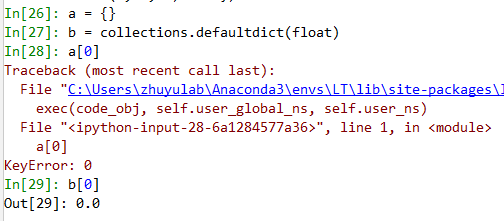如果你访问普通字典（上例中的a）中的未定义的键值时， 会直接报KeyError错误。 但如果是defaultdict，会根据你一开始的参数(float)， 返回一个默认值。 如，我们在中，把float作为输入的参数建立了一个defaultdict b， 那么当我们访问没有定义的b时，会自动返回默认初始化值，float对应的是0.

 def play_n_random_steps(self, count):
for _ in range(count):
action = self.env.action_space.sample()
new_state, reward, is_done, _ = self.env.step(action)
self.rewards[(self.state, action, new_state)] = reward
self.transits[(self.state, action)][new_state] += 1
self.state = self.env.reset() if is_done else new_state


self.rewards这个字典（其实是defaultdict），键值是(当前状态， 动作， 新状态)， 值是这一转换——通过执行该动作从当前状态转换到新状态的reward。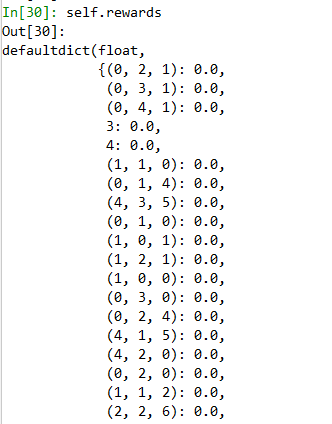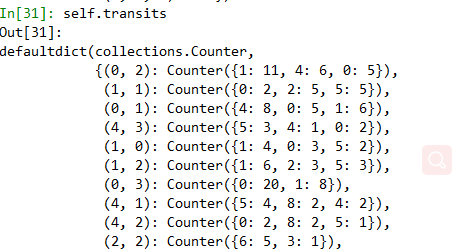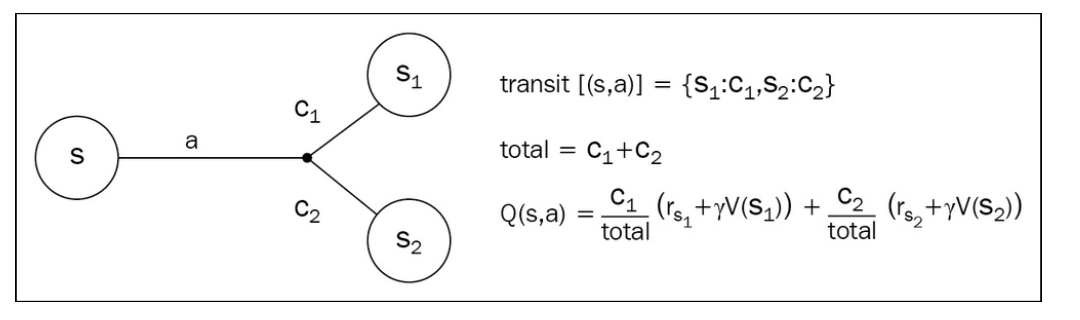def calc_action_value(self, state, action):
target_counts = self.transits[(state, action)]
total = sum(target_counts.values())
action_value = 0.0
for tgt_state, count in target_counts.items():
reward = self.rewards[(state, action, tgt_state)]
action_value += (count / total) * (reward + GAMMA * self.values[tgt_state])
return action_value


    def value_iteration(self):
for state in range(self.env.observation_space.n):
state_values = [self.calc_action_value(state, action)
for action in range(self.env.action_space.n)]
self.values[state] = max(state_values)


   def select_action(self, state):
best_action, best_value = None, None
for action in range(self.env.action_space.n):
action_value = self.calc_action_value(state, action)
if best_value is None or best_value < action_value:
best_value = action_value
best_action = action
return best_action

def play_episode(self, env):
total_reward = 0.0
state = env.reset()
while True:
action = self.select_action(state)
new_state, reward, is_done, _ = env.step(action)
self.rewards[(state, action, new_state)] = reward
self.transits[(state, action)][new_state] += 1
total_reward += reward
if is_done:
break
state = new_state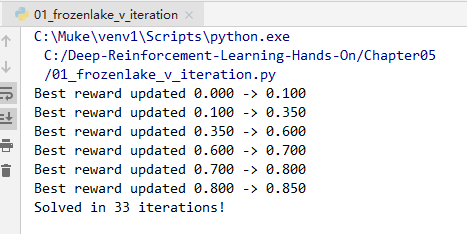## 总结

01-111232

#### 强化学习/动态规划：贝尔曼方程的解读 Bellman Equation & 贝尔曼方程组 / 贝尔曼最优方程

12-192万+

#### 强化学习——值函数与Bellman方程05-131717

#### 强化学习入门介绍--马尔可夫决策过程、最优化原理、贝尔曼方程

09-091941

#### 增强学习（二）：Q-Learning与深度学习结合

04-241902

#### 强化学习：贝尔曼方程

05-31166

#### python强化学习之Q-learning算法

01-071万+

#### 强化学习之二：Q-Learning原理及表与神经网络的实现（Q-Learning with Tables and Neural Networks）

07-086748

#### 贝尔曼方程

06-05244

#### 强化学习：Q-learning与DQN（Deep Q Network）

01-021万+

#### Q学习和深度Q学习（DQN）论文笔记©️2020 CSDN 皮肤主题: 大白 设计师: CSDN官方博客点击重新获取扫码支付1.余额是钱包充值的虚拟货币，按照1:1的比例进行支付金额的抵扣。
2.余额无法直接购买下载，可以购买VIP、C币套餐、付费专栏及课程。余额充值